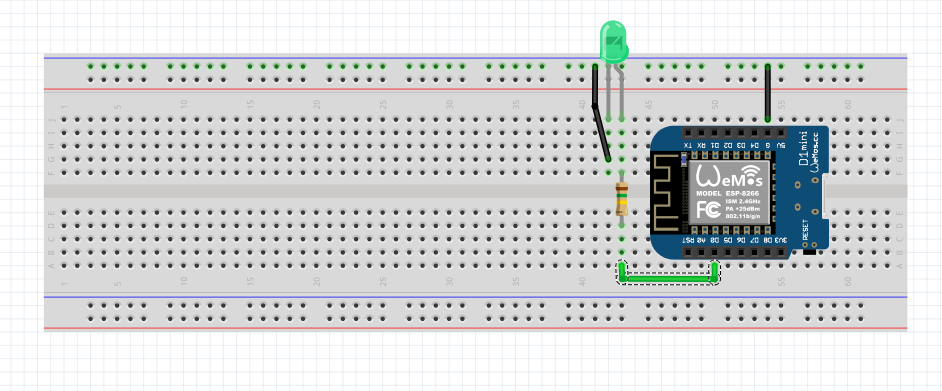# B4R TutorialCheck the solderings of a WeMos D1 Mini

When you buy a WeMos it doesn't come assembled with the pins soldered. You will have to solder the pins. In the following schematic and code you will find a way to check the solderings of GPIO pins. All you have to do is just move the jumper wire from every GPIO Pin to the next (D0->D5->D6->D7->D8->D1->D2->D3->D4). If the led flashes when the looper code goes to your pin the soldering is fine.B4X:
``````#Region Project Attributes
#AutoFlushLogs: True
#CheckArrayBounds: True
#StackBufferSize: 300
#End Region

Sub Process_Globals
'These global variables will be declared once when the application starts.
'Public variables can be accessed from all modules.
Public Serial1 As Serial

Dim p(9) As Pin
Dim d1 As D1Pins

Dim ii As Int = 0
End Sub

Private Sub AppStart
Serial1.Initialize(9600)
Log("AppStart")

p(0).Initialize(d1.D0, p(0).MODE_OUTPUT)
p(0).DigitalWrite(False)

p(1).Initialize(d1.D1, p(1).MODE_OUTPUT)
p(1).DigitalWrite(False)

p(2).Initialize(d1.D2, p(2).MODE_OUTPUT)
p(2).DigitalWrite(False)

p(3).Initialize(d1.D3, p(3).MODE_OUTPUT)
p(3).DigitalWrite(False)

p(4).Initialize(d1.D4, p(4).MODE_OUTPUT)
p(4).DigitalWrite(False)

p(5).Initialize(d1.D5, p(5).MODE_OUTPUT)
p(5).DigitalWrite(False)

p(6).Initialize(d1.D6, p(6).MODE_OUTPUT)
p(6).DigitalWrite(False)

p(7).Initialize(d1.D7, p(7).MODE_OUTPUT)
p(7).DigitalWrite(False)

p(8).Initialize(d1.D8, p(8).MODE_OUTPUT)
p(8).DigitalWrite(False)

End Sub

Sub FlashGreenLight2Times
ii = ii + 1
If ii = 9 Then ii = 0
Log("D", ii)

For jj = 0 To 1
p(ii).DigitalWrite(True)
Delay(200)
p(ii).DigitalWrite(False)
Delay(200)
Next
End Sub``````

Last edited:
•Johan Schoeman, Erel and Kiffi

Replies
7
Views
1K
Replies
6
Views
5K
Replies
2
Views
4K
B4R Question WeMos D1 R2 & DHT22
Replies
14
Views
5K